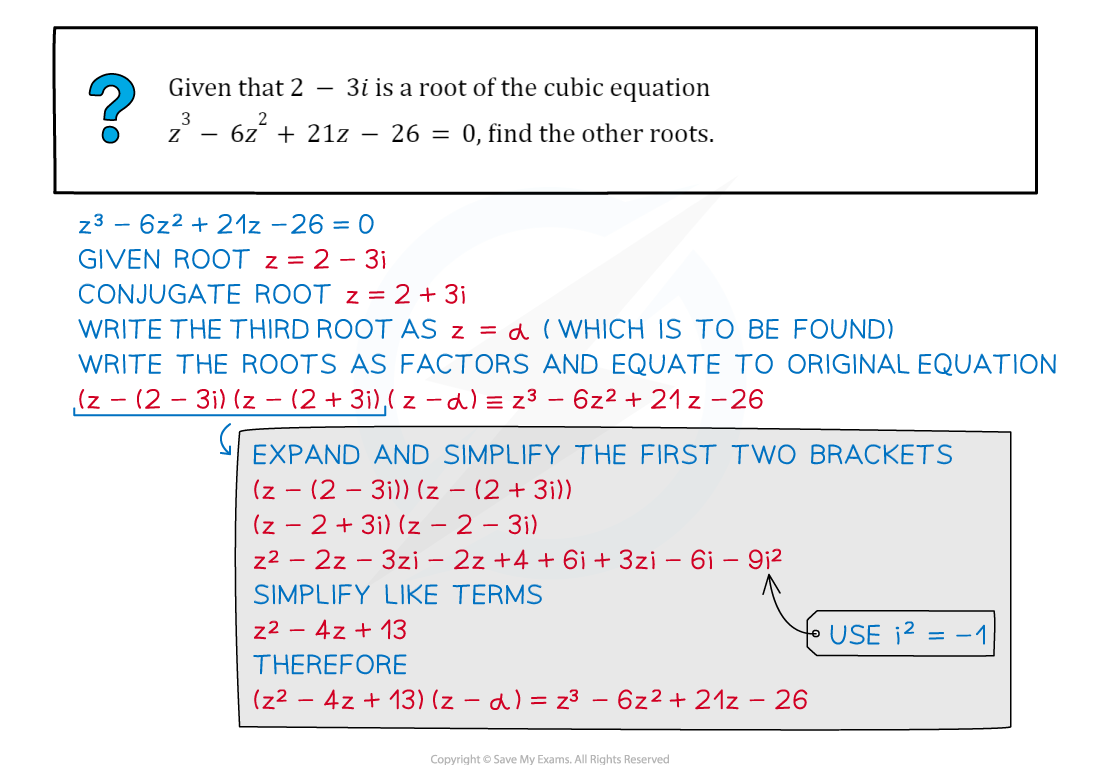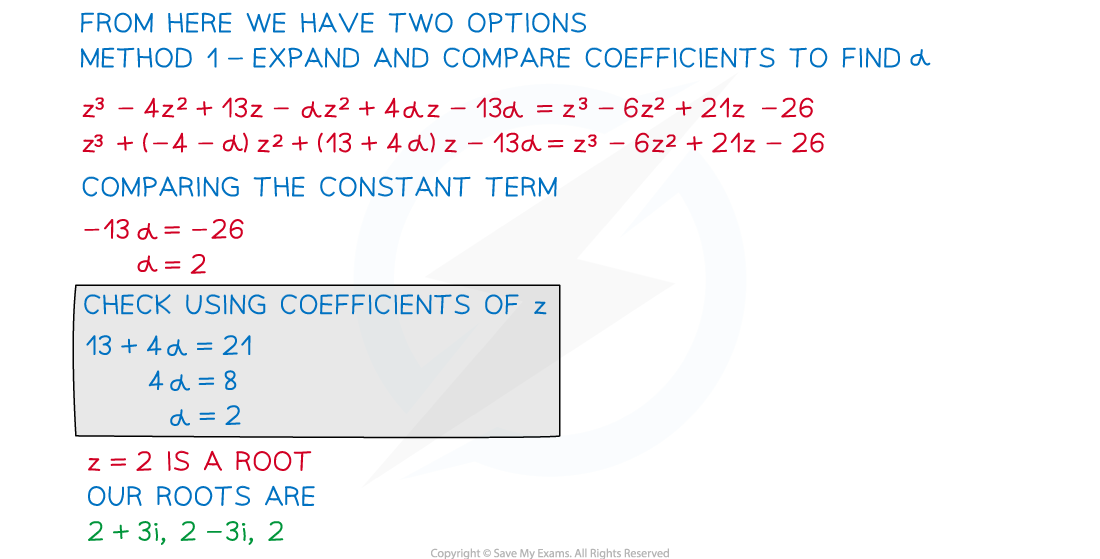# CIE A Level Maths: Pure 3复习笔记8.1.4 Complex Roots of Polynomials

### Complex Roots of Quadratics

#### What are complex roots?

• Complex numbers provide solutions for quadratic equations which have no real roots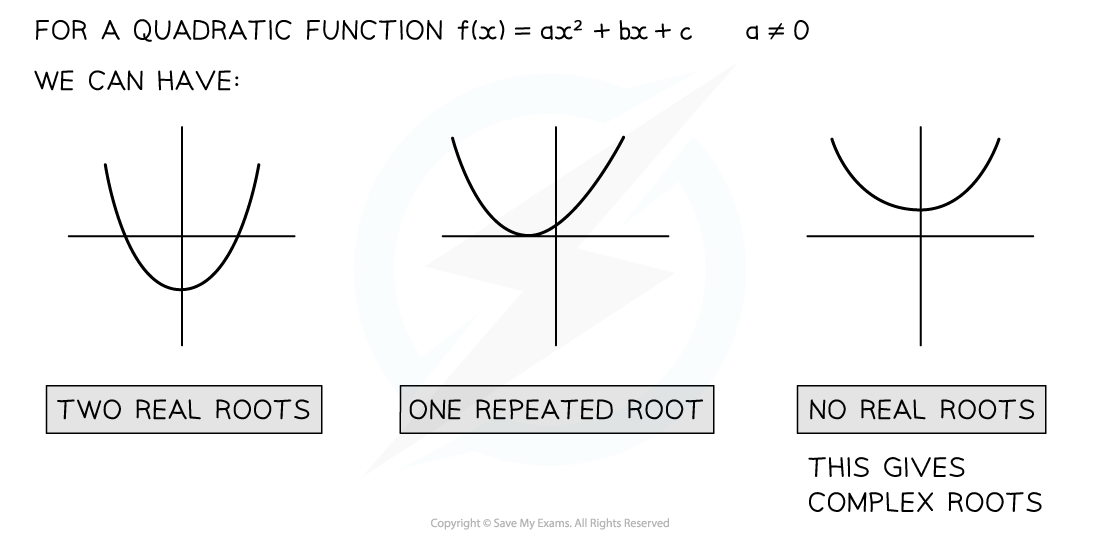• Complex roots occur when solving a quadratic with a negative discriminant
• This leads to square rooting a negative number

#### How do we solve a quadratic equation with complex roots?

• We solve an equation with complex roots in the same way we solve any other quadratic equations
• If in the form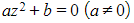we can rearrange to solve
• If in the form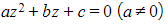we can complete the square or use the quadratic formula
• We use the property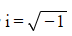along with a manipulation of surds
•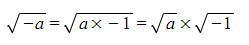• When the coefficients of the quadratic equation are real, complex roots occur in complex conjugate pairs
• If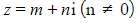is a root of a quadratic with real coefficients then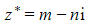is also a root
• When the coefficients of the quadratic equation are non-real, the solutions will not be complex conjugates
• To solve these use the quadratic formula

#### How do we find a quadratic equation given a complex root?

• We can find the equation of the form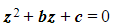if you are given a complex root in the form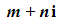• We know that the complex conjugate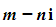is another root,
• This means that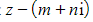and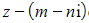are factors of the quadratic equation
• Therefore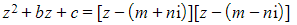• Writing this as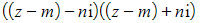will speed up expanding
• Expanding and simplifying gives us a quadratic equation where b and c are real numbers

#### Worked Example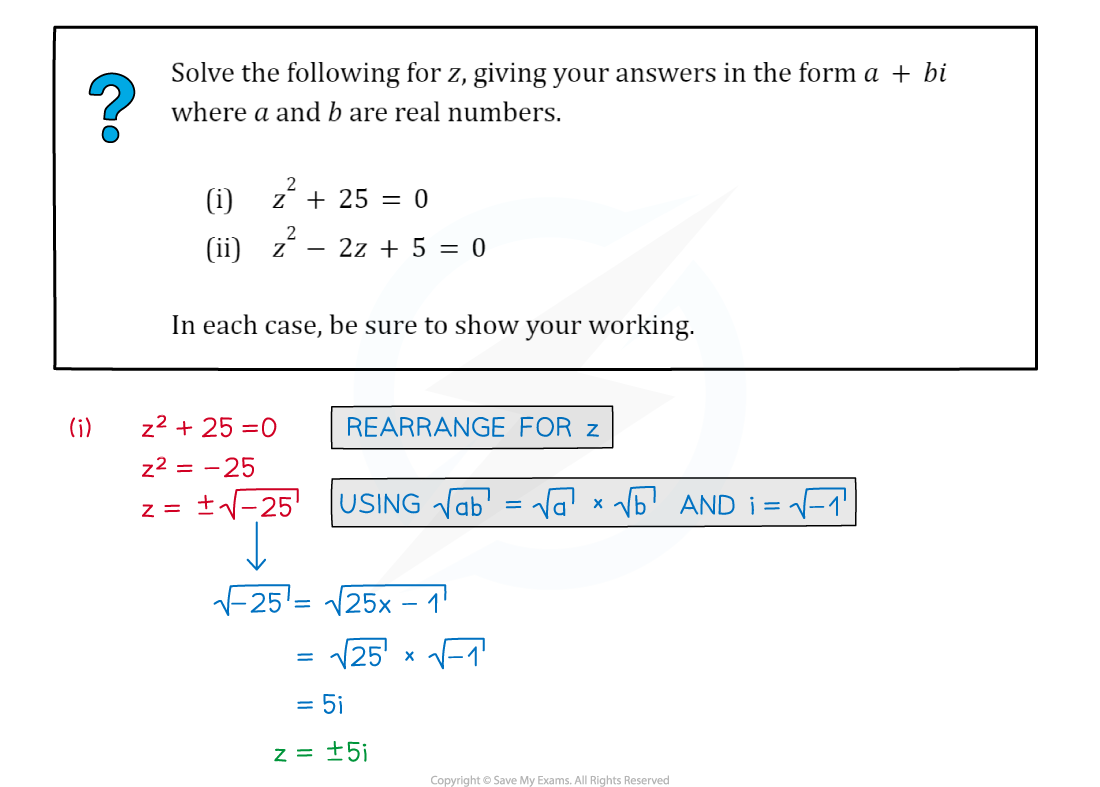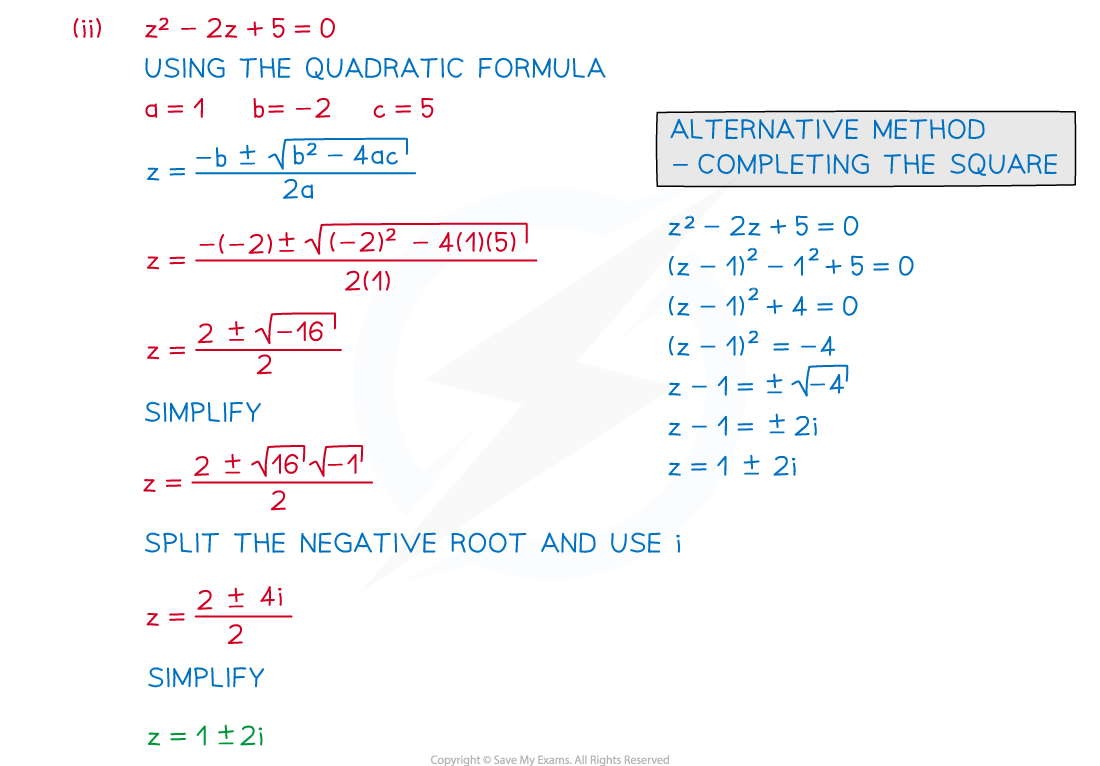#### Exam Tip

• Once you have your final answers you can check your roots are correct by substituting your solutions back into the original equation.
• You should get 0 if correct! [Note: 0 is equivalent to 0 + 0i]

### Complex Roots of Cubics & Quartics

#### How many roots should a polynomial have?#### How do we solve a cubic equation with complex roots?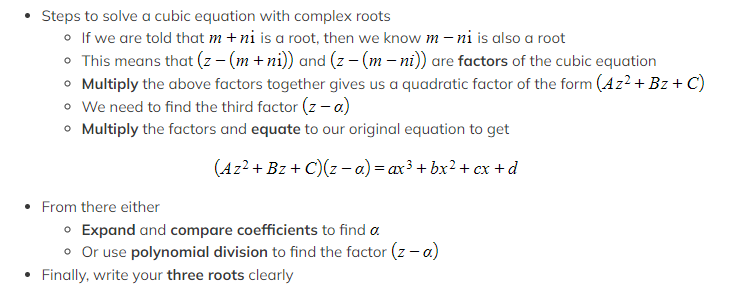#### How do we solve a quartic equation with complex roots?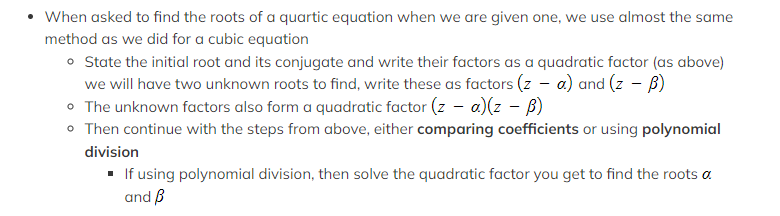#### How do we solve cubic/quartic equations with unknown coefficients?#### Worked Example